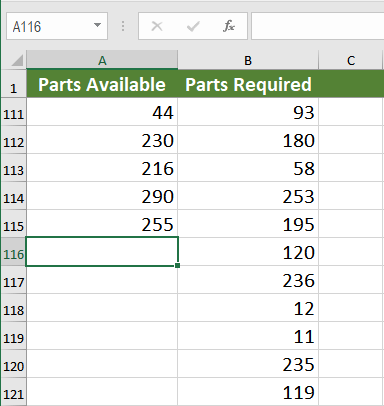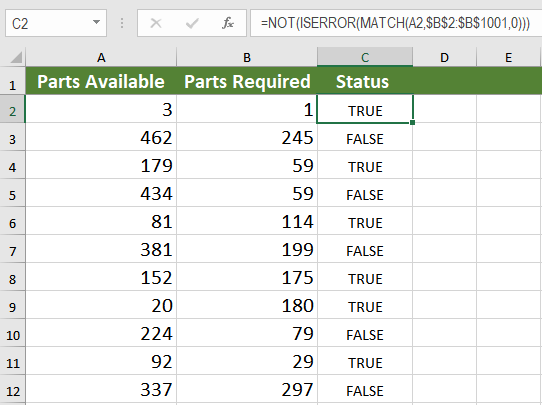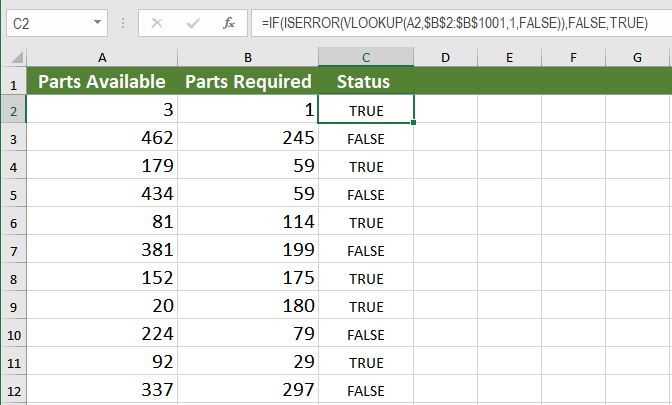Get instant live expert help with Excel or Google Sheets“My Excelchat expert helped me in less than 20 minutes, saving me what would have been 5 hours of work!”

#### Post your problem and you'll get expert help in seconds

Your message must be at least 40 characters
Our professional experts are available now. Your privacy is guaranteed.

# Check If One Column Value Exists in Another ColumnRead time: 20 minutes

When you need to check if one column value exists in another column in Excel, there are several options. One of the most important features in Microsoft Excel is lookup and reference. The VLOOKUP, HLOOKUP, INDEX and MATCH functions can make life a lot easier in terms of looking for a match.

In this tutorial, we will see the use of VLOOKUP and INDEX/MATCH to check if one values from one column exist in another column.

## Check if one column value exists in another column

In the following example, you will work with automobile parts inventory data set. Column A has the parts available, and column B has all the parts needed. Column A has 115 entries, and column B has 1001 entries. We will discuss a couple of ways to match the entries in column A with the ones in column B. Column C will output “True” if there is a match, and “False” if there isn’t.### Check if one column value exists in another column using MATCH

You can use the MATCH() function to check if the values in column A also exist in column B. MATCH() returns the position of a cell in a row or column. The syntax for MATCH() is `=MATCH(lookup_value, lookup_array, [match_type])`. Using MATCH, you can look up a value both horizontally and vertically.

### Example using MATCH

To solve the problem in the previous example with MATCH(), you need to follow the following steps:

1. Select cell C2 by clicking on it.
2. Insert the formula in`"=NOT(ISERROR(MATCH(A2,\$B\$2:\$B\$1001,0)))”` the formula bar.
3. Press Enter to assign the formula to C2.4. Drag the formula down to the other cells in column C clicking and dragging the little “+” icon on the bottom-right of C2.

Excel will match the entries in column A with the ones in column B. If there is a match, it will return the row number. The NOT() and ISERROR() functions check for an error which would be and column C will show “True” for a match and “False” if it is not a match.

### Check if one column value exists in another column using VLOOKUP

VLOOKUP is one of the lookup, and reference functions in Excel and Google Sheets used to find values in a specified range by “row.” It compares them row-wise until it finds a match. In this tutorial, we will look at how to use VLOOKUP on multiple columns with multiple criteria. The syntax for VLOOKUP is `=VLOOKUP(value, table_array, col_index,[range_lookup])`.

### Example using VLOOKUP

You can check if the values in column A exist in column B using VLOOKUP.

1. Select cell C2 by clicking on it.
2. Insert the formula in`“=IF(ISERROR(VLOOKUP(A2,\$B\$2:\$B\$1001,1,FALSE)),FALSE,TRUE)”` the formula bar.
3. Press Enter to assign the formula to C2.4. Drag the formula down to the other cells in column C clicking and dragging the little “+” icon on the bottom-right of C2.

This will allow Excel to look up all the values in column A and match them with the values of column B. Column C will now show “True” if the corresponding cell in column A has a match in column B, “False” if it does not have a match.

### MATCH vs. VLOOKUP – Which is Better?

Between MATCH and VLOOKUP, the better option is MATCH. Not only the formula is simpler, but MATCH is also faster than VLOOKUP when it comes to performance.

MATCH uses a dynamic column reference whereas VLOOKUP uses a static one. If you were to add more columns, with VLOOKUP, you would distort the results. But with MATCH you can easily change the reference when inserting or deleting columns.

Another issue with VLOOKUP is that the lookup value needs to be on the leftmost column of the array, which is not applicable for MATCH. In the previous example, If we had to check if Column C values exist in column A, VLOOKUP would have provided an #N/A error.

Excel has some very effective functions when it comes to checking if values in one column exist in another column. One of these functions is MATCH. It returns the row number based on the lookup value from an array. Another such function is VLOOKUP. It returns the value based on a lookup value from a static array.

When it comes to which function is faster, MATCH is the winner. For its dynamic reference and easy to change array references, MATCH is preferred to check if values in one column also exist in another column.

Most of the time, the problem you need is probably more complex than just simply checking if a value exists in a column. If you want to save hours of researching and frustration, try our Excel Live Chat service! Our Excel experts are available 24/7 to answer any Excel question you have on the spot. The first question is free.

Are you still looking for help with the VLOOKUP function? View our comprehensive round-up of VLOOKUP function tutorials here.

### Did this post not answer your question? Get a solution from connecting with the expert.Another blog reader asked this question today on Excelchat:
Related blogs## Subscribe to Excelchat.coAnother blog reader asked this question today on Excelchat: